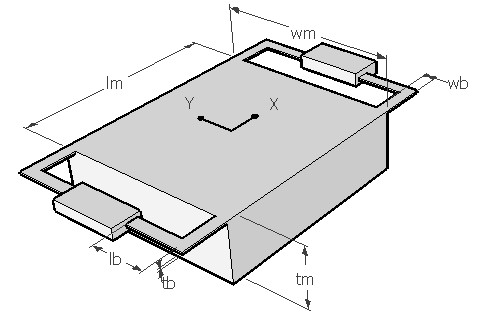BNTFRQ,lb,wb,tb,lm,wm,tm,poi,den,ymod,sel
Vibration frequency of a bent beam suspension

lb            length of beam in µm
wb          width of beam in µm
tb           thickness of beam in µm
lm           length of the mass in µm
wm         width of the mass in µm
tm           thickness of the mass in µm
poi          Poisson's ratio
den        density of the material in kg/m3
ymod     Young's modulus of the material in GPa
sel          number denoting the selected result.
Use 2 for first modal frequencyNotes

The bent beam is formed by two spring segments perpendicular to each other, joined at the corner of the central mass as shown in the picture. The two segments of the beam can have different length, but is assumed to be equal. The mass is suspended on four such bent beams.  A typical structure is as shown above.

This design interface can be used to determine the natural vibration frequency of a bent beam suspension. The vibration frequency for the lowest vibration mode can be estimated. The first mode would move the mass perpendicular to its plane in Z axis.

The plot shows the amplitude frequency relationship for the given suspension for the first mode. It shows the first resonance frequency as a sharp rise in amplitude. Using the cross hair tool, the resonant frequency and the corresponding relative amplitude can be obtained.

Assumptions

-The default material is Silicon with a Young's modulus of 180GPa and Poisson's ratio of 0.3.
-The beam has uniform rectangular cross section.
-The two segments of the beam are equal in length.
-The mass is assumed to be supported on four identical bent beams.
-For determining stiffness of the beam only bending effects are considered.